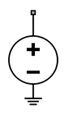# Continuous Wave

Model constant envelope source

• Library:
• RF Blockset / Circuit Envelope / Sources

•## Description

The Continuous Wave block models a constant modulation on a carrier in the RF Blockset™ circuit envelope simulation environment. For an introduction to RF simulation, see the example, Simulate High Frequency Components.

The block implements the relation

`$v\left(t\right)={v}_{0}{e}^{j{\omega }_{k}t}$`

or

`$i\left(t\right)={i}_{0}{e}^{j{\omega }_{k}t}$`

at the carrier ωk, depending on the source type.

## Parameters

expand all

• `Ideal Voltage` — The block simulates a constant voltage envelope v0 at the specified Carrier frequencies. The envelope has real and imaginary parts specified by the Constant in-phase value and Constant quadrature value parameters.

• `Ideal Current` — The block simulates a constant current envelope i0 at the specified Carrier frequencies. The envelope has real and imaginary parts determined by the Constant in-phase value and Constant quadrature value parameters.

• `Power` — The block simulates the constant voltage envelope

`${v}_{0}=2\sqrt{{\text{P}}_{0}\text{Re}\left({Z}_{s}\right)}{e}^{j\frac{\pi }{180}\varphi }$`

where:

• P0 is the value of the Available power parameter

• Zs is the value of the Source impedance (ohms) parameter.

• ϕ is the value of the Angle (degrees) parameter.

In-phase modulations for each of the , specified as a vector of real numbers. Specify the units from the corresponding drop-down list.

#### Dependencies

To enable this parameter, on the Source type tab, choose `Ideal voltage` or ```Ideal current```.

Quadrature modulations for each of the RF circuit carrier frequencies, specified as a vector of real numbers. Specify the units from the corresponding drop-down list.

#### Dependencies

To enable this parameter, on the Source type tab, choose `Ideal voltage` or ```Ideal current```.

Input impedance of source, specified as a positive real number or a complex number. The complex number must contain real and imaginary parts greater than or equal to `1e-18` ohms.

#### Dependencies

To enable this parameter, on the Source type tab, choose `Power`.

Available power at the specified Carrier frequencies, specified as a vector of real numbers. Specify the units from the drop-down list. The default value is `0` `W`.

#### Dependencies

To enable this parameter, on the Source type tab, choose `Power`. The default value is `0` `W`

Phase angle of power waves at the specified Carrier frequencies, specified as a vector of real numbers. The default value is `0` degrees.

#### Dependencies

To enable this parameter, on the Source type tab, choose `Power`.

Carrier frequencies, specified as a vector of real positive numbers. The elements in the carrier frequencies are combinations of fundamental tones and corresponding harmonics in the Configuration block. The default value is `0` `Hz`.

Select this parameter, to add phase noise to your system with continuous wave source.

Phase noise frequency offset, specified as a scalar or vector or matrix with each element units in hertz.

If you specify a matrix, each column should correspond to a non-DC carrier frequency of the CW source. The frequency offset values must be bounded by the envelope bandwidth of the simulation. For more information see, Configuration.

#### Dependencies

To enable this parameter, select Add phase noise.

Phase noise level, specified as a scalar or vector or matrix with element unit in decibel per hertz.

If you specify a matrix, each column should correspond to a non-DC carrier frequency of the CW source. The frequency offset values must be bounded by the envelope bandwidth of the simulation. For more information see, Configuration.

#### Dependencies

To enable this parameter, select Add phase noise.

Select this parameter to automatically calculate impulse response for phase noise. Clear this parameter to manually specify the impulse response duration using Impulse response duration.

Impulse response duration used to simulate phase noise, specified as a scalar in seconds. You cannot specify impulse response if the amplifier is nonlinear.

Note

The phase noise profile resolution in frequency is limited by the duration of the impulse response used to simulate it. Increase this duration to improve the accuracy of the phase noise profile. A warning message appears if the phase noise frequency offset resolution is too high for a given impulse response duration, specifying the minimum duration suitable for the required resolution

#### Dependencies

To set this parameter, first clear Automatically estimate impulse response duration.

Select this option to internally ground and hide the negative terminals. To expose the negative terminals, clear the option. By exposing these terminals, you can connect them to other parts of your model.

By default, this option is selected.